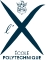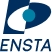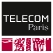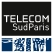Share

# Course Finder

PhD

### Applied Mathematics

Field(s) : Applied mathematics, statistics Mathematics and applications
Specialtie(s) : Applied algebra Applied analysis Numerical analysis Scientific computing Applied geometry Mathematical engineering Financial mathematics Modeling Optimization Applied probability Applied statistics
Duration : 3 years
Language(s) : French English
PhD

### Mathematics at Interfaces

Field(s) : Applied mathematics, statistics Mathematics and applications
Specialtie(s) : Mathematics-economics Mathematics-IT Mathematics-mechanical engineering Mathematics-physics Mathematics-engineering science Mathematics-life sciences
Duration : 3 years
Language(s) : French English
PhD

### Pure Mathematics

Field(s) : Applied mathematics, statistics Mathematics and applications
Specialtie(s) : Algebra Analysis Geometry Logic Probability Statistics
Duration : 3 years
Language(s) : French English